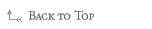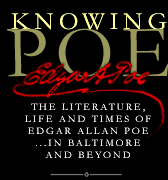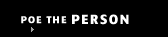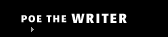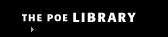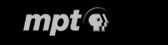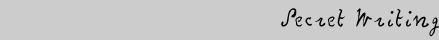Secret Writing 1 2 3 4 5 6 Interactive Secret Writing

5. How ciphers work

There are many different kinds of secret writing and speaking. However, the most common and easiest to break is the substitution cipher. This was the method Poe used a great deal in his writing. Solving a cryptogram like this relies on three things:

• Knowing the language the cryptographer used to make up the cipher
• Knowing that one letter is substituted for another
• Knowing that the letters in the cipher are separated into words

Sometimes a substitution cipher is built around a pattern. Sometimes the letters in the cipher are chosen randomly.

To solve a simple substitution cipher, there are several strategies that you can use:

• The most commonly-used letters today are (in this order):
E  T  N  A  O  R  I  S  H
Knowing this, you can count the number of times a particular letter is used in a cryptogram. For example, if there are more "X"s in the cryptogram than any other letter, "X" might stand for "E" or "T." You can then try out these letters in the cryptogram and see if they make sense.
• The five least-commonly used letters are (in this order):
J  K  Q  X  Z
Knowing this, you can count the number of times a particular letter is used in a cryptogram. For example, if there is only one "M" in the cryptogram, "M" might stand for one of these letters. You can then try out these letters in the cryptogram and see if they make sense.
• There are only two one-word letters in English: A and I
Knowing this, you can look for any characters that have spaces on either side of them. If you find any, you will know that this character is either an "A" or an "I." You can then try out these letters in the cryptogram and see if they make sense.
• The most frequently used two-letter words in English are:
Am  An  As  At
Of  On  Or  Ox
If  In  Is  It
Up  Us
Be  He  Me  We
Do  Go  No  So  To
By  My

Knowing this, you can experiment with the cryptogram. You can substitute any word above for any two-letter combination in the cryptogram and see if it makes sense.
• The most commonly used three letter word in English is the.
Knowing this, you can substitute "the" for any three-letter combination in the puzzle to see if it makes sense. To further explore this, you can substitute the three letters throughout the puzzle to see if it makes sense.
• You can tell a lot from punctuation. An apostrophe indicates a contraction such as I'm, or he's. Knowing this, you can expect that the letter after the apostrophe might be a symbol for "S," "D," or "M." You can expect that the group of words right before a question mark are questions. Try these starting words in the question to see if they make sense: "Who," "What," "Why," "When," "Where," or "How." You can experiment with these combinations to see if they make sense.

Are you ready to try out these theories? Try to solve these cryptograms.